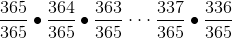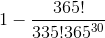Search

# Solution to the Birthday ProblemSolution:

Using the fact that the probabilities of all possible outcome is always equal to 1 (i.e. 100% chance),

P(at least 2 people share the same birthday) + P (No people share the same birthday) = 1

We have to found the probability that there is no people share the same birthday.

Lets do this step by step.

Person A can be born on any given day of the year. The probability of being born any day of the year is 1, or specifically 365/365.

Person B cannot be born on the same day as person A. The probability of not being born on the same day as person A is 364/365.

Person C cannot be born on the same day as person A and B. The probability of not being born on the same day as person A and B is 363/365.

The pattern continues until the last person has a probability (365-29)/365 = 336/365

Multiply all these together, we get the probability that there is no people share the same birthday.Therefore, the probability that at least 2 out of 30 people share the same birthday is*There is around 70.6% chance that 2 or more people share the same birthday in a group of 30!

*Note:

Using factorials, the probability could also be expressed in a more simplified form:which could be entered into a scientific calculator.